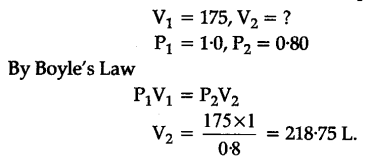# A weather ballon has a volume of 175 L when filled with hydrogen at a pressure of 100 atm

A weather ballon has a volume of 175 L when filled with hydrogen at a pressure of 100 atm. Calculate the volume of the balloon when it rises to a height of 2000 m, where the atmospheric pressure is 0.80 atm. Assume that the temperature is constant